https://doi.org/10.5194/acp-19-10391-2019
https://doi.org/10.5194/acp-19-10391-2019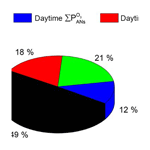# Alkyl nitrates in the boreal forest: formation via the NO3-, OH- and O3-induced oxidation of biogenic volatile organic compounds and ambient lifetimes

Jonathan Liebmann, Nicolas Sobanski, Jan Schuladen, Einar Karu, Heidi Hellén, Hannele Hakola, Qiaozhi Zha, Mikael Ehn, Matthieu Riva, Liine Heikkinen, Jonathan Williams, Horst Fischer, Jos Lelieveld, and John N. Crowley
Abstract

The formation of alkyl nitrates in various oxidation processes taking place throughout the diel cycle can represent an important sink of reactive nitrogen and mechanism for chain termination in atmospheric photo-oxidation cycles. The low-volatility alkyl nitrates (ANs) formed from biogenic volatile organic compounds (BVOCs), especially terpenoids, enhance rates of production and growth of secondary organic aerosol. Measurements of the NO3 reactivity and the mixing ratio of total alkyl nitrates (ΣANs) in the Finnish boreal forest enabled assessment of the relative importance of NO3-, O3- and OH-initiated formation of alkyl nitrates from BVOCs in this environment. The high reactivity of the forest air towards NO3 resulted in reactions of the nitrate radical, with terpenes contributing substantially to formation of ANs not only during the night but also during daytime. Overall, night-time reactions of NO3 accounted for 49 % of the local production rate of ANs, with contributions of 21 %, 18 % and 12 % for NO3, OH and O3 during the day. The lifetimes of the gas-phase ANs formed in this environment were on the order of 2 h due to efficient uptake to aerosol (and dry deposition), resulting in the transfer of reactive nitrogen from anthropogenic sources to the forest ecosystem.

Share
Dates
1 Introduction

Alkyl nitrates (ANs; R−CH2ONO2) are formed in chain-terminating reactions that limit photochemical cycling of organic and HOx radicals and represent an important sink for atmospheric nitrogen (Liu et al., 2012; Lee et al., 2016; Huang et al., 2019). During daytime, ANs are formed in a minor branch of the reaction of NO with organic peroxy radicals (RO2; Lightfoot et al., 1992), which are formed mainly via the OH-initiated oxidation of volatile organic compounds (VOCs):

$\begin{array}{}\text{(R1)}& \mathrm{OH}+\mathrm{RH}\left(+{\mathrm{O}}_{\mathrm{2}}\right)\to {\mathrm{RO}}_{\mathrm{2}},\end{array}$

$\begin{array}{}\text{(R2a)}& {\mathrm{RO}}_{\mathrm{2}}+\mathrm{NO}+M\to {\mathrm{RONO}}_{\mathrm{2}}+M,\text{(R2b)}& {\mathrm{RO}}_{\mathrm{2}}+\mathrm{NO}\to \mathrm{RO}+{\mathrm{NO}}_{\mathrm{2}}.\end{array}$

The yield of ANs in Reactions (R1)–(R2) varies with the structure of the R substituent, temperature and pressure and for small alkyl groups (e.g. R=H, CH3 and C2H5) is generally less than a few percent at 1 bar and 298 K but may increase to values close to 20 % for RO2 formed from the OH initiation of biogenic VOCs (Perring et al., 2013; L. Lee et al., 2014b; IUPAC, 2019).

The reaction with O3 represents an additional sink for biogenic volatile organic compounds (BVOCs; Peräkylä et al., 2014; Yan et al., 2016) which, in the presence of NO, can also lead to the formation of alkyl nitrates. The reaction proceeds by initial addition of O3 to the C=C bond of, for example, a terpene to form a primary ozonide (POZ; Reaction R3) which rapidly decomposes (Reaction R4) via Criegee intermediates to form OH and (in the presence of O2) RO2. The latter reacts via Reactions (R1) and (R2) to form alkyl nitrates:

$\begin{array}{}\text{(R3)}& {\mathrm{O}}_{\mathrm{3}}+R=R\to \mathrm{POZ},\end{array}$

At night-time, OH-radical concentrations are very low, and the NO3 radical, formed by the oxidation of NO2 by ozone (Reaction R5), is the main initiator of oxidation of several classes of VOCs with Reaction (R6a), the dominant pathway for alkyl nitrate formation (Rosen et al., 2004; Crowley et al., 2010; Rollins et al., 2013; Sobanski et al., 2017):

$\begin{array}{}\text{(R5)}& {\mathrm{NO}}_{\mathrm{2}}+{\mathrm{O}}_{\mathrm{3}}\to {\mathrm{NO}}_{\mathrm{3}}+{\mathrm{O}}_{\mathrm{2}},\end{array}$

Reaction (6a) is a composite process involving initial formation of a nitroalkyl radical (via electrophilic addition of NO3 to a C=C double bond) followed by the formation of a nitrooxyperoxy radical (via further addition of O2) which can react with NO, NO3, HO2 or RO2 to form substituted alkyl nitrates (IUPAC, 2019).

The branching ratio to AN formation via NO3 oxidation is generally much larger than that for organic peroxy radicals reacting with NO and, for biogenic VOCs (BVOCs), can approach 80 % (Ng et al., 2017; IUPAC, 2019). The formation of ANs via the degradation of saturated and unsaturated VOCs initiated by NO3, OH and O3 is summarized in Fig. 1.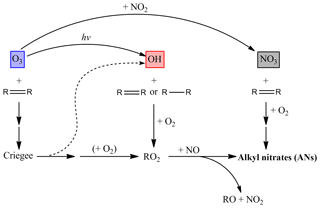Figure 1Schematic diagram illustrating the formation of ANs via VOC degradation initiated by NO3, OH and O3 reactions.

During daytime, the reactions of NO3 with VOCs are often reduced in importance by the rapid photolysis of NO3 and by its reaction with NO (Reactions R7–R9; Wayne et al., 1991):

$\begin{array}{}\text{(R7)}& & {\mathrm{NO}}_{\mathrm{3}}+\mathrm{NO}\to \mathrm{2}{\mathrm{NO}}_{\mathrm{2}},\text{(R8)}& & {\mathrm{NO}}_{\mathrm{3}}+h\mathit{\nu }\to {\mathrm{NO}}_{\mathrm{2}}+\mathrm{O},\text{(R9)}& & {\mathrm{NO}}_{\mathrm{3}}+h\mathit{\nu }\to \mathrm{NO}+{\mathrm{O}}_{\mathrm{2}}.\end{array}$

The fraction (f) of NO3 radicals that undergo reaction with VOCs can be calculated according to Eq. (1):

$\begin{array}{}\text{(1)}& f=\phantom{\rule{0.125em}{0ex}}\frac{{k}_{\mathrm{OTG}}^{{\mathrm{NO}}_{\mathrm{3}}}}{{k}_{\mathrm{OTG}}^{{\mathrm{NO}}_{\mathrm{3}}}+{J}_{{\mathrm{NO}}_{\mathrm{3}}}+\phantom{\rule{0.125em}{0ex}}{k}_{\mathrm{7}}\left[\mathrm{NO}\right]},\end{array}$

where ${k}_{\mathrm{OTG}}^{{\mathrm{NO}}_{\mathrm{3}}}$ is the first-order loss frequency for NO3 reaction with organic trace gases (NO3 reactivity), ${J}_{{\mathrm{NO}}_{\mathrm{3}}}$ is the photolysis rate constant, and [NO]k7 is the NO concentration multiplied by the rate constant for Reaction (R7). Recent measurements of NO3 reactivity in forested regions (Ayres et al., 2015; Liebmann et al., 2018a, b) suggest that, even during the day, a significant fraction of the nitrate radicals generated in Reaction (R5) can react with BVOCs rather than undergoing photolysis or reaction with NO to reform ${\mathrm{NO}}_{x}\left({\mathrm{NO}}_{x}=\mathrm{NO}+{\mathrm{NO}}_{\mathrm{2}}\right)$. During a 2016 field experiment in the boreal forest (IBAIRN – Influence of Biosphere-Atmosphere Interactions on the Reactive Nitrogen budget), we showed that on average more than 20 % of NO3 radicals formed during the day were lost due to reaction with BVOCs (Liebmann et al., 2018a). In this work, we examine the contribution of the NO3-initiated oxidation of VOCs to the formation of ANs both during the daytime and night-time during IBAIRN and compare this to AN formation initiated by reactions of OH and O3. Using calculations of the overall production rate of ANs and measurements of the summed mixing ratio of ANs (ΣANs), we derive a lifetime for ANs in this environment.

2 Measurements

The measurements were made during the IBAIRN field experiment in September 2016 at the “Station for Measuring Forest Ecosystem–Atmosphere Relations II” (SMEAR II) in Hyytiälä (6151 N, 2417 E) in southern Finland. A detailed description of the measurement site can be found elsewhere (Rinne et al., 2005; Lappalainen et al., 2009; Aaltonen et al., 2011). Briefly, the measurement site is located in the boreal forest with mostly biogenic influences. Anthropogenic emissions from two larger cities (Tampere and Jyväskylä) and a local sawmill occasionally impacted the site, the former resulting in an increase in NOX levels and the latter in BVOCs.

The IBAIRN campaign took place in the transition between summer and autumn, with the length of day decreasing from 14 h at the beginning of the campaign to 11 h 5 min at the end. Campaign temperatures, relative humidity, and wind speed and direction can be found in Fig. S1 of the Supplement. During IBAIRN, the diel temperature varied from a night-time minimum of 2 C to a daytime maximum of 20 C, with the nights often being characterized by a strong temperature inversion and a very shallow boundary layer, which resulted in higher monoterpene mixing ratios than during daytime. The relative humidity reached 100 % during many nights with ground-level fog formation. There was no rainfall during the campaign, and the wind was predominantly from north-western directions, with wind speeds never exceeding 5 m s−1. The NOx levels during the entire campaign were low (mean value of 320 pptv), with occasional increases (up to 1.4 ppbv) when the site experienced air masses with trajectories that passed over urban centres. Daily O3 maxima were 30–35 ppbv, with much lower values (5–10 ppbv) on those nights with strong temperature inversions (Liebmann et al., 2018a).

The mixing ratios of OH, NO, NO2, O3, ΣANs and VOCs; the NO3 photolysis frequency; and the NO3 reactivity are required for examining the formation and loss of ANs during IBAIRN. Most instruments used for the data analysis sampled from a common inlet (at 8 m height) on a clearing within the boreal forest. Exceptions were measurement of some organic trace gases and the measurements of actinic flux (see below). As the instruments have been described previously (Liebmann et al., 2018a), we list only the limits of detection (LODs) and 2σ total uncertainty here.

NO was measured using a modified commercial chemiluminescence detector (LOD = 5 pptv in 60 s; 2σ=20 %). O3 was measured by optical absorption (LOD = 1 ppbv in 10 s; 2σ=5 %). NO2 (LOD = 60 pptv in 6 s; 2σ=6 %) and ΣANs (LOD = 40 pptv in 10 min; 2 σ=20 %) were measured using the five-channel, thermal dissociation cavity ring-down spectrometer (TD-CRDS) described in detail by Sobanski et al. (2016). The TD-CRDS instrument also indicated that NO3 levels were less than 1 pptv throughout the campaign.

${J}_{{\mathrm{NO}}_{\mathrm{3}}}$ was calculated from actinic flux measured at 35 m height using a spectral radiometer (Metcon GmbH) and evaluated NO3 cross sections and quantum yields (Burkholder et al., 2015). Ultraviolet-B radiation (280–320 nm) was sampled at 18 m height (Solar Light SL501A radiometer). NO3 reactivity was measured with a recently developed instrument coupling a flow-tube reactor with CRDS (Liebmann et al., 2017, 2018a, b). Isoprene and monoterpenes were measured from a common inlet using a gas chromatograph with an atomic-emission detector (GC-AED; LOD 1 pptv in 20 min; 2σ 14 %), as described in Liebmann et al. (2018a). Organic acids, alcohols, aldehydes and several alkanes were measured on the same clearing but at 1.5 m height, roughly 30 m away from the common inlet using a gas chromatography–mass spectrometer set-up (GC-MS). Details are found in Hellén et al. (2018). OH-radical concentrations were not directly measured during IBAIRN but obtained from a correlation of ground-level OH measurements with ultraviolet-B radiation intensity ([UVB]; in units of W m−2) at this location, with [OH] =5.62×105 [UVB]0.62 (Rohrer and Berresheim, 2006; Petäjä et al., 2009; Hellén et al., 2018). The calculated, ground-level OH concentrations were multiplied by a factor of 2 to take gradients in OH between ground-level and at canopy height into account (Hens et al., 2014). The OH concentrations have an associated uncertainty of ∼50 %.

Real-time measurements of gas- and particle-phase oxidation products formed in the boreal forest were conducted using an Aerodyne high-resolution, long time-of-flight chemical ionization mass spectrometer (HR-L-ToF-CIMS) equipped with iodide (I) reagent ion chemistry (Lopez-Hilfiker et al., 2014, 2015; Lee et al., 2016; Riva et al., 2019). The instrument was coupled to a Filter Inlet for Gases and Aerosols (FIGAERO). Analyses were restricted to ions containing an iodide adduct, which guarantees detection of the parent organic compounds without substantial fragmentation. Iodide CIMS has been described previously and demonstrated high sensitivity towards oxygenated organic compounds, including alkyl nitrates both in the gas and particle phases (Lee et al., 2016). Aerosol surface areas were calculated using data from a differential mobility particle sizer permanently installed at the SMEAR station.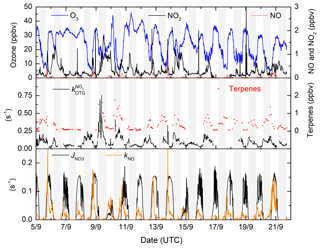Figure 2Time series of the NO3 precursors (NO2 and O3), the NO3 reactivity to organic trace gases (${k}_{\mathrm{OTG}}^{{\mathrm{NO}}_{\mathrm{3}}}$), and the first-order loss constants for its photolysis (${J}_{{\mathrm{NO}}_{\mathrm{3}}}$) and reaction with NO (kNO) during IBAIRN. Grey shaded regions are night-time.

3 Results and discussion

## 3.1 AN production from NO3 reactions with VOCs

In Fig. 2 we display a time series of the NO3 precursors (NO2 and O3) and the NO3 reactivity (${k}_{\mathrm{OTG}}^{{\mathrm{NO}}_{\mathrm{3}}}$) together with the NO3 loss rate constant resulting from its reaction with NO and photolysis. The latter also serves to delineate day (${J}_{{\mathrm{NO}}_{\mathrm{3}}}\ge \mathrm{5}×{\mathrm{10}}^{-\mathrm{4}}$ s−1) and night.

The instantaneous production rate of ANs from the reaction of NO3 with VOCs ($\sum {\mathrm{P}}_{\mathrm{ANs}}^{{\mathrm{NO}}_{\mathrm{3}}}$) is given by

$\begin{array}{}\text{(2)}& \sum {\mathrm{P}}_{\mathrm{ANs}}^{{\mathrm{NO}}_{\mathrm{3}}}={\stackrel{\mathrm{‾}}{\mathit{\alpha }}}^{{\mathrm{NO}}_{\mathrm{3}}}f\left[{\mathrm{O}}_{\mathrm{3}}\right]\left[{\mathrm{NO}}_{\mathrm{2}}\right]{k}_{\mathrm{5}},\end{array}$

where ${\stackrel{\mathrm{‾}}{\mathit{\alpha }}}^{{\mathrm{NO}}_{\mathrm{3}}}$ is an average AN yield. Assuming that all the VOCs responsible for loss of NO3 were identified and quantified, the average yield can be derived (Eq. 3) from VOC-specific values of ${\mathit{\alpha }}_{i}^{{\mathrm{NO}}_{\mathrm{3}}}$ weighted by their relative contribution to ${k}_{\mathrm{OTG}}^{{\mathrm{NO}}_{\mathrm{3}}}$:

$\begin{array}{}\text{(3)}& {\stackrel{\mathrm{‾}}{\mathit{\alpha }}}^{{\mathrm{NO}}_{\mathrm{3}}}=\frac{\sum {\mathit{\alpha }}_{i}^{{\mathrm{NO}}_{\mathrm{3}}}{k}_{i}^{{\mathrm{NO}}_{\mathrm{3}}}\left[{\mathrm{C}}_{i}\right]}{{k}_{\mathrm{OTG}}^{{\mathrm{NO}}_{\mathrm{3}}}},\end{array}$

where [Ci] is the concentration of the specific VOC. The rate constants and branching ratios used to calculate $\sum {\mathrm{P}}_{\mathrm{ANs}}^{{\mathrm{NO}}_{\mathrm{3}}}$ for individual BVOCs can be found in Table S1 of the Supplement. A large selection of VOCs were measured (a listing is given in the caption to Fig. S2), but the five biogenic VOCs listed (α-pinene, β-pinene, carene, limonene and isoprene) accounted for more than 98 % of the attributed NO3 reactivity.

Combining expressions (Eqs. 1–3), we derive the following:

$\begin{array}{}\text{(4)}& \sum {\mathrm{P}}_{\mathrm{ANs}}^{{\mathrm{NO}}_{\mathrm{3}}}=\frac{\sum {\mathit{\alpha }}_{i}^{{\mathrm{NO}}_{\mathrm{3}}}{k}_{i}^{{\mathrm{NO}}_{\mathrm{3}}}\left[{\mathrm{C}}_{i}\right]}{{k}_{\mathrm{OTG}}^{{\mathrm{NO}}_{\mathrm{3}}}+{J}_{{\mathrm{NO}}_{\mathrm{3}}}+\phantom{\rule{0.125em}{0ex}}{k}_{\mathrm{7}}\left[\mathrm{NO}\right]}\left[{\mathrm{O}}_{\mathrm{3}}\right]\left[{\mathrm{NO}}_{\mathrm{2}}\right]{k}_{\mathrm{5}}.\end{array}$

Recognizing that [O3][NO2]k5 and the term ${k}_{\mathrm{OTG}}^{{\mathrm{NO}}_{\mathrm{3}}}+{J}_{{\mathrm{NO}}_{\mathrm{3}}}+\phantom{\rule{0.125em}{0ex}}{k}_{\mathrm{7}}\left[\mathrm{NO}\right]$ are the total NO3 production rates and loss rates, respectively, and that their ratio is the concentration of NO3 in the steady state, [NO3]ss, we can also write

$\begin{array}{}\text{(5)}& \sum {\mathrm{P}}_{\mathrm{ANs}}^{{\mathrm{NO}}_{\mathrm{3}}}={\left[{\mathrm{NO}}_{\mathrm{3}}\right]}_{\mathrm{ss}}\sum {\mathit{\alpha }}_{i}^{{\mathrm{NO}}_{\mathrm{3}}}{k}_{i}^{{\mathrm{NO}}_{\mathrm{3}}}\left[{\mathrm{C}}_{i}\right].\end{array}$

Expression (5) can be used to calculate the production rates of ANs if NO3 measurements are above the detection limit, which despite deployment of sensitive instrumentation was not the case in IBAIRN or in previous campaigns in the boreal forest (Rinne et al., 2012; Crowley et al., 2018). This highlights the advantage of measuring the overall reactivity of NO3 instead of its concentration in highly reactive environments.

As described by Liebmann et al. (2018a) the VOC measurements did not account for the total measured reactivity. The reactivity that could not be attributed (kunattributed; see Eq. 6; on average 30 % at night-time and 60 % during daytime) was therefore treated as stemming from a VOC with an alkyl nitrate yield of 0.7:

$\begin{array}{}\text{(6)}& {k}_{\mathrm{unattributed}}={k}_{\mathrm{OTG}}^{{\mathrm{NO}}_{\mathrm{3}}}-\sum {k}_{i}^{{\mathrm{NO}}_{\mathrm{3}}}\left[{\mathrm{C}}_{i}\right].\end{array}$

A value of 0.7 that was chosen as the unattributed reactivity is likely to be due to highly reactive BVOCs (e.g. terpenes that were not measured or sesquiterpenes; see Liebmann et al., 2018a), which have alkyl nitrate yields between 0.6 and 0.8 (IUPAC, 2019). The uncertainty related to the calculation of $\sum {\mathrm{P}}_{\mathrm{ANs}}^{{\mathrm{NO}}_{\mathrm{3}}}$is estimated at 65 %, with contributions of 50 % from ${k}_{\mathrm{OTG}}^{{\mathrm{NO}}_{\mathrm{3}}}+{J}_{{\mathrm{NO}}_{\mathrm{3}}}+{k}_{\mathrm{7}}$[NO], 18 % from [O3][NO2]k5, 30 % from ${\mathit{\alpha }}_{i}^{{\mathrm{NO}}_{\mathrm{3}}}$, 15 % from ${k}_{i}^{{\mathrm{NO}}_{\mathrm{3}}}$ and 15 % from [Ci].

## 3.2 AN production from OH reactions with VOCs

The rate of production of ANs from the OH-radical-initiated oxidation of VOCs $\left({\mathrm{P}}_{\mathrm{ANs}}^{\mathrm{OH}}$) in the presence of NO can be calculated using Eq. (7):

$\begin{array}{}\text{(7)}& \sum {\mathrm{P}}_{\mathrm{ANs}}^{\mathrm{OH}}=\left[\mathrm{OH}\right]\phantom{\rule{0.125em}{0ex}}\sum {\mathit{\alpha }}_{i}^{{\mathrm{RO}}_{\mathrm{2}}}{k}_{i}^{\mathrm{OH}}\left[{\mathrm{C}}_{i}\right],\end{array}$

where [OH] is the OH concentration, ${\mathit{\alpha }}_{i}^{{\mathrm{RO}}_{\mathrm{2}}}$ is the VOC-specific branching ratio for alkyl nitrate formation (via reaction R2a) from the peroxy radical (RO2) formed, and ${k}_{\mathrm{OTG}}^{\mathrm{OH}}$ is the OH reactivity derived from the VOC concentrations [Ci] and the corresponding rate constant ${k}_{i}^{\mathrm{OH}}$ for reaction with OH.

Organic peroxy radicals formed in Reactions (R1) or (R4a) do not react solely with NO but can also react with hydroperoxyl radicals (Reaction R10) and undergo radical recombination (Reaction R11), each of which reduces the production rate of ANs via Reaction (R2a). They can also isomerize (Reaction R12) to form a more oxidized form of RO2:

$\begin{array}{}\text{(R10)}& & {\mathrm{RO}}_{\mathrm{2}}+{\mathrm{HO}}_{\mathrm{2}}\to \mathrm{ROOH}+{\mathrm{O}}_{\mathrm{2}},\text{(R11)}& & {\mathrm{RO}}_{\mathrm{2}}+{\mathrm{RO}}_{\mathrm{2}}\to \phantom{\rule{0.125em}{0ex}}\text{products},\text{(R12)}& & {\mathrm{RO}}_{\mathrm{2}}\phantom{\rule{0.125em}{0ex}}\left(\text{isomerization}\right)+{\mathrm{O}}_{\mathrm{2}}\to {\mathrm{R}}^{\prime }{\mathrm{O}}_{\mathrm{2}}.\end{array}$

Although the rate constant for some cross- and self-reactions of terpene-derived RO2 is large (Berndt et al., 2018), the organic peroxide products of these reactions have only been observed in very low mixing ratios at this site (Yan et al., 2016), and, following Browne et al. (2013), we neglect the impact of Reaction (R11). We also assume that the peroxy radical formed in Reaction (R12) forms an organic nitrate with the same efficiency as the parent RO2 so that Reaction (R12) can also be neglected. The RO2 formed in Reactions (R1) and (R4a) does not form stable peroxy nitrates in its reaction with NO2, so this RO2-loss process can be safely neglected.

To assess the influence of Reaction (R10), we used the noontime ratio of OH to HO2 radicals derived by Crowley et al. (2018) during a campaign at the same location (${\mathrm{HO}}_{\mathrm{2}}\approx \mathrm{250}×\mathrm{OH}$) to calculate the HO2 concentration during IBAIRN from that of OH (calculated itself from actinic flux; see above). The fraction, β, of the RO2 radicals that react with NO is then

$\begin{array}{}\text{(8)}& \mathit{\beta }=\frac{{k}_{{\mathrm{RO}}_{\mathrm{2}}+\mathrm{NO}}\left[\mathrm{NO}\right]}{{k}_{{\mathrm{RO}}_{\mathrm{2}}+\mathrm{NO}}\left[\mathrm{NO}\right]+\phantom{\rule{0.125em}{0ex}}{k}_{{\mathrm{RO}}_{\mathrm{2}}+{\mathrm{HO}}_{\mathrm{2}}}\left[{\mathrm{HO}}_{\mathrm{2}}\right]}.\end{array}$

β was derived by assuming a generic rate coefficient (based on rate coefficients of known RO2; IUPAC, 2019) of ${k}_{{\mathrm{RO}}_{\mathrm{2}}+\mathrm{NO}}=\phantom{\rule{0.125em}{0ex}}\mathrm{8}×{\mathrm{10}}^{-\mathrm{12}}$ and ${k}_{{\mathrm{RO}}_{\mathrm{2}}+{\mathrm{HO}}_{\mathrm{2}}}=\mathrm{1}×{\mathrm{10}}^{-\mathrm{11}}$ cm3 molecule−1 s−1. Campaign average values of β varied from 0.9 in the early morning (04:00–08:30 UTC) during the breakup of the night-time boundary layer to ≈0.8 later in the day (09:30–16:30 UTC), a slight decrease. In order to account for this competition with HO2 reactions, Eq. (7) can be modified to

$\begin{array}{}\text{(9)}& \sum {\mathrm{P}}_{\mathrm{ANs}}^{\mathrm{OH}}=\phantom{\rule{0.125em}{0ex}}\left[\mathrm{OH}\right]\mathit{\beta }\sum {\mathit{\alpha }}_{i}^{{\mathrm{RO}}_{\mathrm{2}}}{k}_{i}^{\mathrm{OH}}\left[{\mathrm{C}}_{i}\right].\end{array}$

The rate constants and branching ratios used for these calculations can be found in Table S1. The total OH reactivity (${k}_{\mathrm{OTG}}^{\mathrm{OH}}$) was calculated using the measured concentrations of monoterpenes and isoprene, as other VOCs (e.g. aldehydes, acids, alkanes and alkenes) contributed less than 6 % (Fig. S2). The calculated OH reactivity from monoterpenes varied between approximately 0.2 and 2 s−1, with a campaign average of ≈0.3 s−1, in accordance with previous measurements (Hellén et al., 2018). The high value of 7 s−1 on 9 September was associated with large BVOC mixing ratios in air masses originating from the local sawmill. The calculated production rate of ANs via Eq. (9) assumes that all ambient VOCs that result in AN formation were measured. However, previous summertime comparisons of total OH reactivity derived from VOC measurements suggest that a significant fraction of the VOC reacting with OH was not identified (Nölscher et al., 2012) and that this fraction depended on the degree of heat and drought-induced stress, with 58 % of the measured OH reactivity remaining unassigned to individual VOCs during “non-stressed” conditions. As OH-reactivity data are not available during IBAIRN, we cannot assess which fraction of OH reactivity could potentially be missing for the much cooler autumn conditions, but we expect this to be less than the 58 % reported for the warmer summer months. We show below that even if unattributed OH reactivity reaches 50 %, this would not significantly modify our conclusions. We estimate an uncertainty in the term $\sum {\mathrm{P}}_{\mathrm{ANs}}^{\mathrm{OH}}$ of ≈70 %, with contributions of 50 % from [OH], 30 % from β, 15 % from ${k}_{i}^{\mathrm{OH}}$, 30 % from ${\mathit{\alpha }}_{i}^{{\mathrm{RO}}_{\mathrm{2}}}$ and 25 % from [Ci].

## 3.3 AN production from O3 reactions with VOCs

The production rate of alkyl nitrates generated via the reaction of O3 with VOCs ($\sum {\mathrm{P}}_{\mathrm{ANs}}^{{\mathrm{NO}}_{\mathrm{3}}}$) in the presence of NO is described by Eq. (10):

$\begin{array}{}\text{(10)}& \sum {\mathrm{P}}_{\mathrm{ANs}}^{{\mathrm{O}}_{\mathrm{3}}}=\left[{\mathrm{O}}_{\mathrm{3}}\right]\mathit{\beta }\sum {\mathit{\alpha }}_{i}^{{\mathrm{O}}_{\mathrm{3}}}\phantom{\rule{0.125em}{0ex}}{k}_{i}^{{\mathrm{O}}_{\mathrm{3}}}\left[{\mathrm{C}}_{i}\right]{\mathit{\alpha }}_{i}^{{\mathrm{RO}}_{\mathrm{2}}},\end{array}$

where [O3] is the O3 concentration, ${\mathit{\alpha }}_{i}^{{\mathrm{O}}_{\mathrm{3}}}$ is the VOC-specific yield of the RO2 radical formed in Reaction (R4), ${\mathit{\alpha }}_{i}^{{\mathrm{O}}_{\mathrm{3}}}$ is the VOC-specific yield of ANs from RO2+NO and is assumed to be the same as for OH-initiated degradation of the same BVOCs, and ${k}_{\mathrm{OTG}}^{{\mathrm{O}}_{\mathrm{3}}}$ is the O3 reactivity derived from the VOC concentrations [Ci] along with the corresponding rate constant ${k}_{i}^{{\mathrm{O}}_{\mathrm{3}}}$ for reaction with O3. Similar to OH reactions, β is the fraction of RO2 radicals that react with NO rather than with HO2 or with themselves (see above).

The OH radicals that are formed in Reaction (R4a) will react according to Reactions (1)–(2) and also increase the alkyl nitrate yield. However, the calculated OH concentration, based on an empirical correlation of observed [OH] with the ultraviolet-B radiation, contains all OH sources, including Reaction (R4a). In order to avoid double counting this source of OH, the RO2 formed in the Reactions (R4), (R1) and (R2) are not taken into account in Eq. (10). The rate constants and branching ratios used for these calculations can be found in Table S1.

We cannot assess which fraction of O3 reactivity could potentially be missing; hence the obtained production rates have to be considered a lower limit. The calculated O3 reactivity is depicted in Fig. S2. While OH molecules are only abundant in higher concentrations during day, O3 is an important oxidizing agent present during day and night. At night, away from direct sources, the NO mixing ratio is reduced to very low values within a few minutes after sunset due to reaction with O3 to form NO2, and therefore the alkyl nitrate production via this channel becomes insignificant. We used the NO3 photolysis rate to delineate day and night and assumed that in the absence of light (${J}_{{\mathrm{NO}}_{\mathrm{3}}}\mathit{<}\mathrm{5}×{\mathrm{10}}^{-\mathrm{4}}$ s−1) the NO mixing ratios are not enough to support production of alkyl nitrates. The uncertainty in the term $\sum {\mathrm{P}}_{\mathrm{ANs}}^{{\mathrm{O}}_{\mathrm{3}}}$ was estimated to be ∼60 %, with contributions of 5 % from [O3], 30 % from β, 30 % from ${\mathit{\alpha }}_{i}^{{\mathrm{O}}_{\mathrm{3}}}$, 15 % from ${k}_{i}^{{\mathrm{O}}_{\mathrm{3}}}$, 30 % from ${\mathit{\alpha }}_{i}^{{\mathrm{RO}}_{\mathrm{2}}}$ and 15 % from [Ci].

## 3.4 Relative importance of OH-, O3- and NO3-initiated VOC oxidation reactions for AN formation

The NO3 production rate; the OH mixing ratios; and the calculated alkyl nitrate production rates from NO3, OH and O3 are depicted in Fig. 3. In this low-NOx environment, the NO3 production rate is highly correlated with NO2 mixing ratios, while the OH mixing ratio is directly coupled to solar radiation. The rate of production of ANs from ozonolysis of BVOC has a daytime minimum at noon, with maximum values observed in the late afternoon.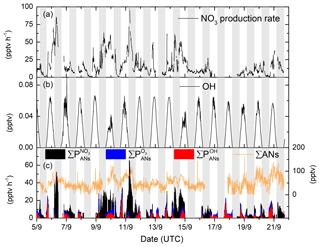Figure 3(a) NO3 radical production rate from reaction of NO2 and O3. (b) calculated OH mixing ratio; see Sect. 2. (c) Production rate of ANs from OH (red), O3 (blue) and NO3 radicals (black) and the ΣAN mixing ratio (orange line).

The largest production rates of ANs are observed at night-time via NO3 reactions though $\sum {\mathrm{P}}_{\mathrm{ANs}}^{{\mathrm{NO}}_{\mathrm{3}}}$, which is highly variable, with values between ≈5 and 65 pptv h−1. In contrast, daytime production via OH ($\sum {\mathrm{P}}_{\mathrm{ANs}}^{\mathrm{OH}}$) is rather reproducible, with maximum values of about 21 pptv h−1 at local noon. On cloudy days (e.g. 10 and 15 September), the OH production rate is reduced significantly, and at the same time the NO3 photolysis rate decreases so that its reactions with VOCs become more important. Both effects combine to enhance the rate of AN generation via NO3 over that of OH. $\sum {\mathrm{P}}_{\mathrm{ANs}}^{{\mathrm{O}}_{\mathrm{3}}}$ can reach up to 16 pptv h−1 in the late afternoon but is usually between 3 and 5 pptv h−1. Figure 3 also plots the time series of ΣANs during the campaign. The mixing ratios of ΣANs are generally very low (generally < 100 pptv) and are sometimes at (or close to) the instrument's limit of detection.

The campaign-averaged contribution of OH, O3 and NO3 to the production of ANs during IBAIRN is displayed as a diel profile in Fig. 4a. The night-time generation of ANs formed via NO3 reaction with BVOCs maximizes at ≈20 pptv h−1 (at ≈19:00), which can be compared to the maximum value of just 10 pptv  h−1 from OH reactions and 6 pptv h−1 from O3 reactions during the day. As almost all NO3 formed in this environment will react with a BVOC at night-time, the production rate of ANs is not sensitive to the mixing ratios of BVOCs. The peak in the night-time production rate of ANs at 19:00 UTC coincides with large O3 and NO2 mixing ratios (Fig. 4b), with the reduction of both O3 and NO2 between ∼20:00 and midnight (UTC) resulting in the decrease in $\sum {\mathrm{P}}_{\mathrm{ANs}}^{{\mathrm{NO}}_{\mathrm{3}}}$ during the night, though changes in relative concentrations of the terpenes may also play a role. The daytime generation of ANs from NO3 reactions is significant and at times approaches 50 % of the overall rate of AN formation.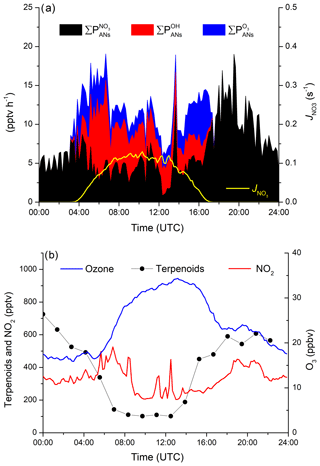Figure 4(a) Diel profiles (means) of the AN production rates for VOC initiated oxidation by the OH radical ($\mathrm{\Sigma }{P}_{\mathrm{AN}}^{\mathrm{OH}}$), O3 ($\mathrm{\Sigma }{P}_{\mathrm{AN}}^{{\mathrm{O}}_{\mathrm{3}}}$) and the NO3 radicals ($\mathrm{\Sigma }{P}_{\mathrm{AN}}^{{\mathrm{NO}}_{\mathrm{3}}}$) and the photolysis rate constant of NO3 (yellow line) to distinguish in between night and day. (b) Diel profiles (means) of the mixing ratios of O3, NO2 and total terpenoids.

The total contributions of OH-, O3- and NO3-induced alkyl nitrate formation over the whole campaign are summarized in pie-chart form in Fig. 5. In total, 51 % of ANs were formed during the day, with 21 % initiated by NO3, 18 % initiated by OH chemistry and 12 % initiated by O3 chemistry. At night-time the AN production rate (exclusively via NO3-initiated reactions) contributes 49 %.

So far we have assumed that the measured VOCs account for the entire reactivity of the OH radical. If, for example, 50 % of the OH reactivity were not accounted for by the measured VOCs (see Sect. 3.2), the contribution of OH would increase from 18 % to 31 %, with the contributions of NO3 decreasing to 18 % (day) and 41 % (night). The contribution of O3 would decrease to 10 %. This does not change the conclusion that NO3 reactions contribute substantially not only at night-time but also to daytime AN formation in this environment.

Ideally, when comparing the relative contributions of daytime and night-time processes to AN formation, we also need to consider the relative volumes of air through which the chemistry is taking place. A very rough estimation of how variations in the boundary-layer height impact the results can be gained by scaling the alkyl nitrate production rates by the height of the fully developed boundary layer during IBAIRN (570 m during the day and 34 m during the night; Hellén et al., 2018) and by considering the relative duration of day (13 h) and night (11 h). Inclusion of a deeper boundary layer by a factor of ≈17 during the day results in drastic shift in the relative roles of NO3 versus OH and O3, with only 6 % of the total boundary-layer alkyl nitrate production being initiated by NO3 reactions at night-time compared to 39 % during the day. By comparison, the daytime, OH-initiated formation of alkyl nitrates accounts for 33 %, whereas O3 accounts for 22 % of the total. These very rough estimates can only be considered illustrative of the potential effects, as they are biased by the inherent assumption that there are no vertical gradients in OH or NO3 reactivity during day or night, which is not the case (Eerdekens et al., 2009; Nölscher et al., 2012; Liebmann et al., 2018a; Zha et al., 2018) and also assumes that the daytime boundary layer reaches its fully developed height immediately after dawn. The true values will depend on the details of mixing within and development of the boundary layer and will lie between the two sets of calculations.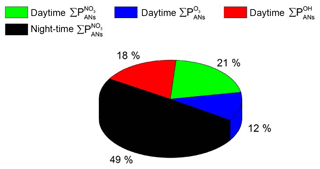Figure 5Contribution of NO3-, OH- and O3-initiated degradation of VOCs to the overall formation of alkyl nitrates.

As illustrated in Fig. 3, the ΣAN mixing ratio reached maximum values of only ∼100 pptv despite production terms of 40 pptv h−1, indicating that the lifetimes of the ANs are relatively short. If this is the case, the local concentration of ANs are largely decoupled from the effects of long-range transport (timescales of days), and the AN lifetime (τANs) can be can be calculated using a steady-state approach via (Eq. 11):

$\begin{array}{}\text{(11)}& {\mathit{\tau }}_{\mathrm{ANs}}=\frac{\left[\mathrm{ANs}\right]}{\sum {\mathrm{P}}_{\mathrm{ANs}}},\end{array}$

where ΣPANs is the total production rate (initiated by NO3, OH and O3). The campaign-averaged, diel variation in the AN mixing ratio and ∑PANs are given in Fig. 6a. A plot of ΣANs versus ∑PANs using the 1 h averages from the diel profiles, is displayed in Fig. 6b, with daytime data represented by the red data points and night-time data by the black data points. Within the overall uncertainty represented by the error bars, there is no significant difference between the daytime and night-time data, with a linear fit through all the data indicating a lifetime of $\approx \mathrm{2}±\mathrm{3}$ h or a loss rate constant of $\mathrm{5.6}×{\mathrm{10}}^{-\mathrm{4}}$ s−1. The intercept of ∼24 pptv ANs can be attributed to small, possibly mono-functional alkyl nitrates that have longer lifetimes and which therefore could have been transported to the site rather than generated locally (Clemitshaw et al., 1997; Romer et al., 2016).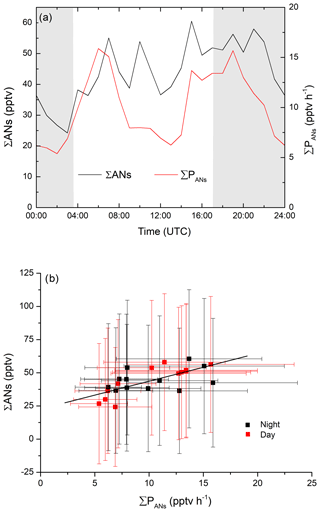Figure 6(a) Diel profiles of production rate and mixing ratios of ANs during IBAIRN. (b) Plot of ΣAN mixing ratios versus the total production rate from NO3-, OH- and O3-initiated VOC oxidation during the day (06:00–15:00 UTC; black dots) and night-time (18:00–03:00 UTC; red dots). The slope of the linear fit to the data (York fit; errors in both axes considered; black line) indicates a lifetime of 2±3 h. The error bars correspond to the total uncertainty as described in the text.

We now examine the potential reasons for the short lifetime of ANs at this location. ANs are generally thought to react inefficiently with O3, OH and NO3, and low rates of photolysis mean that their lifetimes are likely to be controlled largely by dry deposition and/or heterogeneous hydrolysis on aerosol or hydrometeors (Browne et al., 2013). Known exceptions are some ANs formed from isoprene, which can react with OH and/or be photolysed with lifetimes on the order of an hour (Müller et al., 2014; Xiong et al., 2016). During IBAIRN, isoprene-derived ANs were however only a small fraction of the total, and, in the absence of kinetic and/or photochemical data for terpenes, we disregard gas-phase chemical loss processes.

In a well-mixed daytime boundary layer the deposition velocity (Vdep) is equal to kdepHBL, where HBL is the boundary layer height and kdep is the first-order loss rate constant due to deposition. Taking a value of Vdep ∼1–2 cm s−1 for ANs (Farmer and Cohen, 2008; Nguyen et al., 2015) and an average, noontime boundary layer height of 570 m, we derive an average lifetime with respect to deposition of 8–16 h, substantially longer than that observed. Conversely, a deposition velocity of ∼8 cm s−1 would result in a lifetime of ∼2 h, consistent with our observations.

The contribution of loss of ANs via heterogeneous uptake to sub-micron aerosol (khet) can be assessed via Eq. (12):

$\begin{array}{}\text{(12)}& {k}_{\mathrm{het}}=\frac{\mathit{\gamma }\phantom{\rule{0.125em}{0ex}}\stackrel{\mathrm{‾}}{c}\phantom{\rule{0.125em}{0ex}}A}{\mathrm{4}},\end{array}$

where γ is the uptake coefficient, A is the aerosol surface area density (in cm2 cm−3) and $\stackrel{\mathrm{‾}}{c}$ is the average thermal velocity (in cm s−1). The mean aerosol surface area observed during IBAIRN was $\mathrm{2}×{\mathrm{10}}^{-\mathrm{7}}$ cm2 cm−3 (range $\mathrm{0.4}×{\mathrm{10}}^{-\mathrm{7}}$ cm2 cm−3 to $\mathrm{6}×{\mathrm{10}}^{-\mathrm{7}}$ cm2 cm−3; see Fig. S3). For a typical C10 alkyl nitrate derived from monoterpene oxidation such as C10H14NO7 (Yan et al., 2016; Lee et al., 2018), $\stackrel{\mathrm{‾}}{c}$ is ∼15 000 cm s−1 at 290 K. The average uptake coefficient required to reproduce a loss rate constant for ANs of $\mathrm{5.6}×{\mathrm{10}}^{-\mathrm{4}}$ s−1 would then be 0.8, which is orders of magnitude larger than the values of 10−3–10−4 reported for water-soluble organics (Wu et al., 2015; Crowley et al., 2018). However, the high-molecular-weight, biogenically derived ANs in the boreal forest having low vapour pressures and transferring via condensation to existing particles are likely to be important. In this case transfer to the particle phase may be controlled by diffusion and accommodation, and the effective uptake efficiency could be much larger. Once transferred to the particle phase, a short lifetime with respect to hydrolysis to HNO3 will result in permanent (irreversible) loss of the AN from the gas phase (Browne et al., 2013; Bean and Hildebrandt Ruiz, 2016; Lee et al., 2016, 2018; Romer et al., 2016; Zare et al., 2018) and eventually to deposition (as inorganic nitrate) to the plant surfaces and forest floor. The deposition of nitrate thus represents the last step in a sequence of biological and photochemical processes that enables, via emission of BVOCs, the trapping by the biosphere of reactive, gas-phase NOX of largely anthropogenic origin and thus the transfer of nitrogen back to the ecosystem. Especially in nitrogen-poor environments (e.g. at high latitudes) this represents an important route for plant and forest fertilization (Fowler et al., 2013; Huang et al., 2019).

If, as suggested, the gas-phase organic nitrates formed via the three pathways above are indeed transferred to the aerosol phase on short timescales, we would expect to find some correlation between the total production rate and aerosol nitrate content, either as organic nitrate or, following hydrolysis, HNO3. Aerosol mass spectrometer (AMS) measurements of aerosol composition were available for a ∼10 d period during the campaign, and we show a plot of AMS nitrate versus the total AN production rate in Fig. 7. The AMS-nitrate mass loading (µg m−3) was converted to a mixing ratio using an atomic mass of 63 (i.e. assuming HNO3).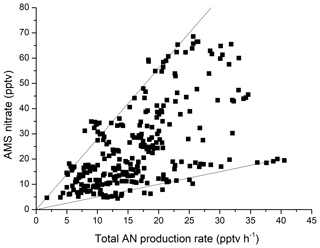Figure 7Total particle nitrate (AMS) versus the total production rate of ANs from reactions of NO3, OH and O3 with BVOCs. The solid lines are upper and lower bounds with slopes of ∼2.8 and 0.5 h, respectively.

Figure 7 illustrates that the highest nitrate aerosol content is correlated with high AN production rates, with the approximately zero intercept indicating that the formation of particulate nitrate independent of AN formation is negligible. As the formation of ANs requires NOX, this is not surprising, as in the absence of NOX, particulate nitrate formation would also tend to zero. However, a plot of AMS nitrate versus NOX over the same period (Fig. S4) is more scattered, supporting the argument that the combination of BVOC oxidation in the presence of NOX (i.e. AN formation) is a major source of aerosol nitrate in this environment.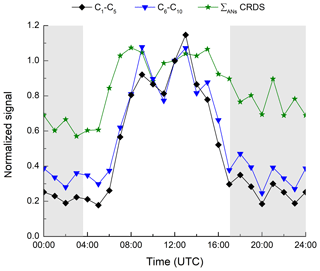Figure 8Relative diel profile of HR-L-ToF-CIMS signals attributed to C1–C5 and C6–C10 organic nitrates and comparison with ΣANs measured by the TD-CRDS. Data normalized at 12:00 UTC.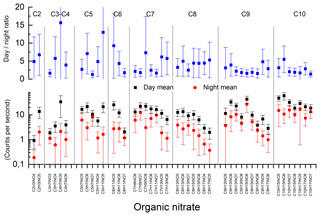Figure 9Campaign mean signals for organic nitrates measured by the HR-L-ToF-CIMS. The HR-L-ToF-CIMS was not calibrated; thus only the raw signal (counts per second) at each mass is given.

By taking up ANs, particles effectively integrate the AN production term over time, and the slopes of the solid lines in Fig. 7 represent integration times of 2.8 h (upper bound) and 0.5 h (lower bound) factored by the efficiency of uptake. The latter may be related to several factors that control the transfer of gas-phase ANs to the particle phase, including relative humidity, temperature, available aerosol surface area, release of HNO3 back to the gas phase and (competitively) dry deposition. Colour coding the data in Fig. 7 for various parameters (temperature; relative humidity; aerosol surface area; or other particle properties like ammonium, sulfate and organic mass) revealed that the larger slopes are associated with higher organic content (Fig. S4), which in turn is expected to be associated with more aged aerosol. No trend was found in parameters such as temperature and relative humidity, suggesting that their influence on the transfer of ANs to the particle phase is weak. We cannot explore the role of dry deposition of ANs in detail but suggest that this is unlikely to vary enough to induce the observed variation in the slopes observed in Fig. 7. If we assume 100 % transfer of ANs to the particle phase, the integration time (upper bound to the data in Fig. 7) represents the maximum lifetime (with respect to deposition) of 2.8 h for the aerosol.

Our TD-CRDS instrument measures the total concentration of ANs without speciation and therefore does not allow us to investigate whether ANs formed via OH-, O3- or NO3-initiated degradation of BVOCs have different lifetimes. The molecular composition of a total of 45 organic nitrates (C1–C10, O3–O8, N1) was identified using the HR-L-ToF-CIMS, which is known to be sensitive towards alkyl nitrates (Lee et al., 2014b, 2016). In the following discussion we therefore consider the masses to be associated mainly with alkyl nitrates (and not, for example, peroxy nitrates). Neither the absolute nor the relative sensitivity (between different alkyl nitrates) is known, and the following discussion is necessarily qualitative in nature. Assuming equal sensitivity across the mass spectrum for the HR-L-ToF-CIMS measurements in IBAIRN (B. H. Lee et al., 2014; Lee et al., 2016), we find that in total only 10 % of the organic nitrates were C5, whereas C6–C10 accounted for 85 % of the signal. This is indicated in Fig. S4.

In Fig. 8, we compare the TD-CRDS measurement of the ΣANs measurement to the signal of the HR-L-ToF-CIMS, the latter separated into C1–C5 and C6–C10 species. As the HR-L-ToF-CIMS data are available as counts only, we have normalized to the values at 12:00 UTC. The C1–C5 and C6–C10 trace gases identified by the HR-L-ToF-CIMS display very similar diel cycles, with daytime maxima and low values at night-time, as also seen for the ΣANs data. The HR-L-ToF-CIMS data do however indicate a much more pronounced diel cycle, which is related to the different positions of the inlets of the instruments. Whereas the TD-CRDS sampled at a height of 8.5 m above the ground, the HR-L-ToF-CIMS inlet was located at a height of just 1.5 m. As mentioned already, high relative humidity was frequently accompanied by ground-level fog at night-time during IBAIRN, which would have significantly impacted the HR-L-ToF-CIMS dataset, resulting in lower mean night-time signals. In addition, we cannot rule out that this difference is due to the different HR-L-ToF-CIMS sensitivity to daytime and night-time ANs.

Figure 9 plots the daytime and night-time campaign mean signal at each organic nitrate mass throughout the campaign. C9 and C10 nitrates provided the greatest signals on average, followed by C7 and C8. As seen from the diel average (Fig. 8), the organic-nitrate signals were significantly larger during the day than at night-time, with the lowest day-to-night ratios being found among the C9 and C10 species and the largest day–night ratios found for C5 nitrates and smaller. The C5 organic nitrates (and smaller) are likely to stem from isoprene chemistry, whereas C6 nitrates and larger nitrates are likely to be products of terpene oxidation (Lee et al., 2016). Our observations are broadly consistent with the study of Huang et al. (2019) in a mixed isoprene–terpene forest, which observed daytime concentration maxima for C5 species and night-time maxima for C10. A more detailed comparison with Huang et al. (2019) is however difficult, owing to very different isoprene-to-terpene emission rates, which in the IBAIRN campaign strongly favoured terpenes (Liebmann et al., 2018a).

4 Conclusions

During the IBAIRN campaign in the boreal forest in southern Finland (5–22 September 2016), alkyl nitrate formation was dominated by the reaction of NO3 radicals with monoterpenes, both during the daytime and night-time, with smaller contributions from both OH- and O3-initiated oxidation of BVOCs. This result highlights the important role of daytime NO3 chemistry (with respect to organic nitrate formation) in this environment. The short average lifetime of ≈2 h for the total alkyl nitrates (ΣANs) indicates efficient uptake to existing particles and/or deposition.

These observations of efficient daytime production of gas-phase ANs from NO3 chemistry and short night-time lifetimes are entirely consistent with the results from recent studies at the IBAIRN site by Lee et al. (2018), who found that organic nitrates previously designated as resulting from night-time processing of BVOCS (Yan et al., 2016) were also present during daytime. In addition, they found relatively few organics with the “night-time” character in the gas phase compared to the aerosol phase, indicating efficient transfer of gas-phase organic nitrates to the particle phase at night-time, likely aided by low temperatures and high relative humidity. We found no significant change in the production rate of ANs in transition from summer to autumn, though the short duration of the campaign and variability in temperature and insolation would mask such effects.

Data availability

The Max Planck Institute data used in this study are archived with Zenodo at https://doi.org/10.5281/zenodo.3254828 (Crowley and Fischer, 2019). Depending on agreement with the IBAIRN data protocol, the data will be available for external users from August 2019.

Supplement

Author contributions

JonL was responsible for the NO3-reactivity measurements and interpretation during IBAIRN and, with contributions from JNC, HF and JosL, wrote the paper. NS was responsible for the CRDS measurements of ANs, NO3 and NO2. JS was responsible for the O3 and J-value measurements. HF was responsible for the NO measurements. EK, JW, HeH and HaH were responsible for the VOC and BVOC measurements. QZ and MR were responsible for the HR-L-ToF-CIMS measurements of ANs. The IBAIRN campaign was conceived and organized by JNC and ME.

Competing interests

The authors declare that they have no conflict of interest.

Acknowledgements

We would like to thank Uwe Parchatka for the provision of NO measurements and the excellent technical support from Janne Levula and the team at Hyytiälä.

Financial support

This research has been supported by ENVRIplus of the IBAIRN campaign.

The article processing charges for this open-access
publication were covered by the Max Planck Society.

Review statement

This paper was edited by Jacqui Hamilton and reviewed by two anonymous referees.

References

Aaltonen, H., Pumpanen, J., Pihlatie, M., Hakola, H., Hellén, H., Kulmala, L., Vesala, T., and Bäck, J.: Boreal pine forest floor biogenic volatile organic compound emissions peak in early summer and autumn, Agr. Forest Meteorol., 151, 682–691, https://doi.org/10.1016/j.agrformet.2010.12.010, 2011.

Ayres, B. R., Allen, H. M., Draper, D. C., Brown, S. S., Wild, R. J., Jimenez, J. L., Day, D. A., Campuzano-Jost, P., Hu, W., de Gouw, J., Koss, A., Cohen, R. C., Duffey, K. C., Romer, P., Baumann, K., Edgerton, E., Takahama, S., Thornton, J. A., Lee, B. H., Lopez-Hilfiker, F. D., Mohr, C., Wennberg, P. O., Nguyen, T. B., Teng, A., Goldstein, A. H., Olson, K., and Fry, J. L.: Organic nitrate aerosol formation via ${\mathrm{NO}}_{\mathrm{3}}^{+}$ biogenic volatile organic compounds in the southeastern United States, Atmos. Chem. Phys., 15, 13377–13392, https://doi.org/10.5194/acp-15-13377-2015, 2015.

Bean, J. K. and Hildebrandt Ruiz, L.: Gas–particle partitioning and hydrolysis of organic nitrates formed from the oxidation of α-pinene in environmental chamber experiments, Atmos. Chem. Phys., 16, 2175–2184, https://doi.org/10.5194/acp-16-2175-2016, 2016.

Berndt, T., Mender, B., Scholz, W., Fischer, L., Herrmann, H., Kulmala, M., and Hansel, A.: Accretion Product Formation from Ozonolysis and OH Radical Reaction of alpha-Pinene: Mechanistic Insight and the Influence of Isoprene and Ethylene, Environ. Sci. Technol., 52, 11069–11077, https://doi.org/10.1021/acs.est.8b02210, 2018.

Browne, E. C., Min, K. E., Wooldridge, P. J., Apel, E., Blake, D. R., Brune, W. H., Cantrell, C. A., Cubison, M. J., Diskin, G. S., Jimenez, J. L., Weinheimer, A. J., Wennberg, P. O., Wisthaler, A., and Cohen, R. C.: Observations of total RONO2 over the boreal forest: NOx sinks and HNO3 sources, Atmos. Chem. Phys., 13, 4543–4562, https://doi.org/10.5194/acp-13-4543-2013, 2013.

Burkholder, J. B., Sander, S. P., Abbatt, J., Barker, J. R., Huie, R. E., Kolb, C. E., Kurylo, M. J., Orkin, V. L., Wilmouth, D. M., and Wine, P. H.: Chemical Kinetics and Photochemical Data for Use in Atmospheric Studies, Evaluation No. 18, JPL Publication 15-10, Jet Propulsion Laboratory, Pasadena, available at: http://jpldataeval.jpl.nasa.gov, 2015.

Clemitshaw, K. C., Williams, J., Rattigan, O. V., Shallcross, D. E., Law, K. S., and Anthony Cox, R.: Gas-phase ultraviolet absorption cross-sections and atmospheric lifetimes of several C2–C5 alkyl nitrates, J. Photoch. Photobio. A, 102, 117–126, 1997.

Crowley, J. N. and Fischer, H.: IBAIRN data (Boreal forest, Hyytiälä Sept. 2016) Max-Planck-Institut, Mainz, https://zenodo.org/record/3254828#.XVQHfkHRaUk, last access: 14 August 2019.

Crowley, J. N., Schuster, G., Pouvesle, N., Parchatka, U., Fischer, H., Bonn, B., Bingemer, H., and Lelieveld, J.: Nocturnal nitrogen oxides at a rural mountain-site in south-western Germany, Atmos. Chem. Phys., 10, 2795–2812, https://doi.org/10.5194/acp-10-2795-2010, 2010.

Crowley, J. N., Pouvesle, N., Phillips, G. J., Axinte, R., Fischer, H., Petäjä, T., Nölscher, A., Williams, J., Hens, K., Harder, H., Martinez-Harder, M., Novelli, A., Kubistin, D., Bohn, B., and Lelieveld, J.: Insights into HOx and ROx chemistry in the boreal forest via measurement of peroxyacetic acid, peroxyacetic nitric anhydride (PAN) and hydrogen peroxide, Atmos. Chem. Phys., 18, 13457–13479, https://doi.org/10.5194/acp-18-13457-2018, 2018.

Eerdekens, G., Yassaa, N., Sinha, V., Aalto, P. P., Aufmhoff, H., Arnold, F., Fiedler, V., Kulmala, M., and Williams, J.: VOC measurements within a boreal forest during spring 2005: on the occurrence of elevated monoterpene concentrations during night time intense particle concentration events, Atmos. Chem. Phys., 9, 8331–8350, https://doi.org/10.5194/acp-9-8331-2009, 2009.

Farmer, D. K. and Cohen, R. C.: Observations of HNO3, ΣAN, ΣPN and NO2 fluxes: evidence for rapid HOx chemistry within a pine forest canopy, Atmos. Chem. Phys., 8, 3899–3917, https://doi.org/10.5194/acp-8-3899-2008, 2008.

Fowler, D., Pyle, J. A., Raven, J. A., and Sutton, M. A.: The global nitrogen cycle in the twenty-first century: introduction, Philos. T. R. Soc. B, 368, 20130165, https://doi.org/10.1098/rstb.2013.0165, 2013.

Hellén, H., Praplan, A. P., Tykkä, T., Ylivinkka, I., Vakkari, V., Bäck, J., Petäjä, T., Kulmala, M., and Hakola, H.: Long-term measurements of volatile organic compounds highlight the importance of sesquiterpenes for the atmospheric chemistry of a boreal forest, Atmos. Chem. Phys., 18, 13839–13863, https://doi.org/10.5194/acp-18-13839-2018, 2018.

Hens, K., Novelli, A., Martinez, M., Auld, J., Axinte, R., Bohn, B., Fischer, H., Keronen, P., Kubistin, D., Nolscher, A. C., Oswald, R., Paasonen, P., Petaja, T., Regelin, E., Sander, R., Sinha, V., Sipila, M., Taraborrelli, D., Ernest, C. T., Williams, J., Lelieveld, J., and Harder, H.: Observation and modelling of HOx radicals in a boreal forest, Atmos. Chem. Phys., 14, 8723–8747, https://doi.org/10.5194/acp-14-8723-2014, 2014.

Huang, W., Saathoff, H., Shen, X., Ramisetty, R., Leisner, T., and Mohr, C.: Chemical Characterization of Highly Functionalized Organonitrates Contributing to Night-Time Organic Aerosol Mass Loadings and Particle Growth, Environ. Sci. Technol., 53, 1165–1174, https://doi.org/10.1021/acs.est.8b05826, 2019.

IUPAC: Task Group on Atmospheric Chemical Kinetic Data Evaluation, edited by: Ammann, M., Cox, R. A., Crowley, J. N., Herrmann, H., Jenkin, M. E., McNeill, V. F., Mellouki, A., Rossi, M. J., Troe, J., and Wallington, T. J., available at: http://iupac.pole-ether.fr/index.html, last access: May 2019.

Lappalainen, H. K., Sevanto, S., Bäck, J., Ruuskanen, T. M., Kolari, P., Taipale, R., Rinne, J., Kulmala, M., and Hari, P.: Day-time concentrations of biogenic volatile organic compounds in a boreal forest canopy and their relation to environmental and biological factors, Atmos. Chem. Phys., 9, 5447–5459, https://doi.org/10.5194/acp-9-5447-2009, 2009.

Lee, B. H., Lopez-Hilfiker, F. D., Mohr, C., Kurten, T., Worsnop, D. R., and Thornton, J. A.: An Iodide-Adduct High-Resolution Time-of-Flight Chemical-Ionization Mass Spectrometer: Application to Atmospheric Inorganic and Organic Compounds, Environ. Sci. Technol., 48, 6309–6317, https://doi.org/10.1021/es500362a, 2014a.

Lee, B. H., Mohr, C., Lopez-Hilfiker, F. D., Lutz, A., Hallquist, M., Lee, L., Romer, P., Cohen, R. C., Iyer, S., Kurten, T., Hu, W. W., Day, D. A., Campuzano-Jost, P., Jimenez, J. L., Xu, L., Ng, N. L., Guo, H. Y., Weber, R. J., Wild, R. J., Brown, S. S., Koss, A., de Gouw, J., Olson, K., Goldstein, A. H., Seco, R., Kim, S., McAvey, K., Shepson, P. B., Starn, T., Baumann, K., Edgerton, E. S., Liu, J. M., Shilling, J. E., Miller, D. O., Brune, W., Schobesberger, S., D'Ambro, E. L., and Thornton, J. A.: Highly functionalized organic nitrates in the southeast United States: Contribution to secondary organic aerosol and reactive nitrogen budgets, P. Natl. Acad. Sci. USA, 113, 1516–1521, https://doi.org/10.1073/pnas.1508108113, 2016.

Lee, B. H., Lopez-Hilfiker, F. D., D'Ambro, E. L., Zhou, P., Boy, M., Petäjä, T., Hao, L., Virtanen, A., and Thornton, J. A.: Semi-volatile and highly oxygenated gaseous and particulate organic compounds observed above a boreal forest canopy, Atmos. Chem. Phys., 18, 11547–11562, https://doi.org/10.5194/acp-18-11547-2018, 2018.

Lee, L., Wooldridge, P. J., Gilman, J. B., Warneke, C., de Gouw, J., and Cohen, R. C.: Low temperatures enhance organic nitrate formation: evidence from observations in the 2012 Uintah Basin Winter Ozone Study, Atmos. Chem. Phys., 14, 12441–12454, https://doi.org/10.5194/acp-14-12441-2014, 2014b.

Liebmann, J. M., Schuster, G., Schuladen, J. B., Sobanski, N., Lelieveld, J., and Crowley, J. N.: Measurement of ambient NO3 reactivity: design, characterization and first deployment of a new instrument, Atmos. Meas. Tech., 10, 1241–1258, https://doi.org/10.5194/amt-10-1241-2017, 2017.

Liebmann, J. M., Karu, E., Sobanski, N., Schuladen, J., Ehn, M., Schallhart, S., Quéléver, L., Hellen, H., Hakola, H., Hoffmann, T., Williams, J., Fischer, H., Lelieveld, J., and Crowley, J. N.: Direct measurement of NO3 radical reactivity in a boreal forest, Atmos. Chem. Phys., 18, 3799–3815, https://doi.org/10.5194/acp-18-3799-2018, 2018a.

Liebmann, J. M., Muller, J. B. A., Kubistin, D., Claude, A., Holla, R., Plass-Dülmer, C., Lelieveld, J., and Crowley, J. N.: Direct measurements of NO3 reactivity in and above the boundary layer of a mountaintop site: identification of reactive trace gases and comparison with OH reactivity, Atmos. Chem. Phys., 18, 12045–12059, https://doi.org/10.5194/acp-18-12045-2018, 2018b.

Lightfoot, P. D., Cox, R. A., Crowley, J. N., Destriau, M., Hayman, G. D., Jenkin, M. E., Moortgat, G. K., and Zabel, F.: Organic peroxy radicals – kinetics, spectroscopy and tropospheric chemistry, Atmos. Environ. A, 26, 1805–1961, 1992.

Liu, S., Shilling, J. E., Song, C., Hiranuma, N., Zaveri, R. A., and Russell, L. M.: Hydrolysis of Organonitrate Functional Groups in Aerosol Particles, Aerosol Sci. Tech., 46, 1359–1369, https://doi.org/10.1080/02786826.2012.716175, 2012.

Lopez-Hilfiker, F. D., Mohr, C., Ehn, M., Rubach, F., Kleist, E., Wildt, J., Mentel, Th. F., Lutz, A., Hallquist, M., Worsnop, D., and Thornton, J. A.: A novel method for online analysis of gas and particle composition: description and evaluation of a Filter Inlet for Gases and AEROsols (FIGAERO), Atmos. Meas. Tech., 7, 983–1001, https://doi.org/10.5194/amt-7-983-2014, 2014.

Lopez-Hilfiker, F. D., Mohr, C., Ehn, M., Rubach, F., Kleist, E., Wildt, J., Mentel, Th. F., Carrasquillo, A. J., Daumit, K. E., Hunter, J. F., Kroll, J. H., Worsnop, D. R., and Thornton, J. A.: Phase partitioning and volatility of secondary organic aerosol components formed from α-pinene ozonolysis and OH oxidation: the importance of accretion products and other low volatility compounds, Atmos. Chem. Phys., 15, 7765–7776, https://doi.org/10.5194/acp-15-7765-2015, 2015.

Müller, J.-F., Peeters, J., and Stavrakou, T.: Fast photolysis of carbonyl nitrates from isoprene, Atmos. Chem. Phys., 14, 2497–2508, https://doi.org/10.5194/acp-14-2497-2014, 2014.

Ng, N. L., Brown, S. S., Archibald, A. T., Atlas, E., Cohen, R. C., Crowley, J. N., Day, D. A., Donahue, N. M., Fry, J. L., Fuchs, H., Griffin, R. J., Guzman, M. I., Herrmann, H., Hodzic, A., Iinuma, Y., Jimenez, J. L., Kiendler-Scharr, A., Lee, B. H., Luecken, D. J., Mao, J., McLaren, R., Mutzel, A., Osthoff, H. D., Ouyang, B., Picquet-Varrault, B., Platt, U., Pye, H. O. T., Rudich, Y., Schwantes, R. H., Shiraiwa, M., Stutz, J., Thornton, J. A., Tilgner, A., Williams, B. J., and Zaveri, R. A.: Nitrate radicals and biogenic volatile organic compounds: oxidation, mechanisms, and organic aerosol, Atmos. Chem. Phys., 17, 2103–2162, https://doi.org/10.5194/acp-17-2103-2017, 2017.

Nguyen, T. B., Crounse, J. D., Teng, A. P., Clair, J. M. S., Paulot, F., Wolfe, G. M., and Wennberg, P. O.: Rapid deposition of oxidized biogenic compounds to a temperate forest, P. Natl. Acad. Sci. USA, 112, E392–E401, https://doi.org/10.1073/pnas.1418702112, 2015.

Nölscher, A. C., Williams, J., Sinha, V., Custer, T., Song, W., Johnson, A. M., Axinte, R., Bozem, H., Fischer, H., Pouvesle, N., Phillips, G., Crowley, J. N., Rantala, P., Rinne, J., Kulmala, M., Gonzales, D., Valverde-Canossa, J., Vogel, A., Hoffmann, T., Ouwersloot, H. G., Vilà-Guerau de Arellano, J., and Lelieveld, J.: Summertime total OH reactivity measurements from boreal forest during HUMPPA-COPEC 2010, Atmos. Chem. Phys., 12, 8257–8270, https://doi.org/10.5194/acp-12-8257-2012, 2012.

Peräkylä, O., Vogt, M., Tikkanen, O. P., Laurila, T., Kajos, M. K., Rantala, P. A., Patokoski, J., Aalto, J., Yli-Juuti, T., Ehn, M., Sipila, M., Paasonen, P., Rissanen, M., Nieminen, T., Taipale, R., Keronen, P., Lappalainen, H. K., Ruuskanen, T. M., Rinne, J., Kerminen, V. M., Kulmala, M., Back, J., and Petaja, T.: Monoterpenes' oxidation capacity and rate over a boreal forest: temporal variation and connection to growth of newly formed particles, Boreal Environ. Res., 19, 293–310, 2014.

Perring, A. E., Pusede, S. E., and Cohen, R. C.: An observational perspective on the atmospheric impacts of alkyl and multifunctional nitrates on ozone and secondary organic aerosol, Chem. Rev., 113, 5848–5870, https://doi.org/10.1021/cr300520x, 2013.

Petäjä, T., Mauldin, III, R. L., Kosciuch, E., McGrath, J., Nieminen, T., Paasonen, P., Boy, M., Adamov, A., Kotiaho, T., and Kulmala, M.: Sulfuric acid and OH concentrations in a boreal forest site, Atmos. Chem. Phys., 9, 7435–7448, https://doi.org/10.5194/acp-9-7435-2009, 2009.

Rinne, J., Ruuskanen, T. M., Reissell, A., Taipale, R., Hakola, H., and Kulmala, M.: On-line PTR-MS measurements of atmospheric concentrations of volatile organic compounds in a European boreal forest ecosystem, Boreal Environ. Res., 10, 425–436, 2005.

Rinne, J., Markkanen, T., Ruuskanen, T. M., Petäjä, T., Keronen, P., Tang, M. J., Crowley, J. N., Rannik, Ü., and Vesala, T.: Effect of chemical degradation on fluxes of reactive compounds – a study with a stochastic Lagrangian transport model, Atmos. Chem. Phys., 12, 4843–4854, https://doi.org/10.5194/acp-12-4843-2012, 2012.

Riva, M., Heikkinen, L., Bell, D. M., Peräkylä, O., Zha, Q., Schallhart, S., Rissanen, M. P., Imre, D., Petäjä, T., Thornton, J. A., Zelenyuk, A., and Ehn, M.: Chemical transformations in monoterpene-derived organic aerosol enhanced by inorganic composition, NPJ Clim. Atmos. Sci., 2, 2, https://doi.org/10.1038/s41612-018-0058-0, 2019.

Rohrer, F. and Berresheim, H.: Strong correlation between levels of tropospheric hydroxyl radicals and solar ultraviolet radiation, Nature, 442, 184–187, https://doi.org/10.1038/nature04924, 2006.

Rollins, A. W., Pusede, S., Wooldridge, P., Min, K. E., Gentner, D. R., Goldstein, A. H., Liu, S., Day, D. A., Russell, L. M., Rubitschun, C. L., Surratt, J. D., and Cohen, R. C.: Gas/particle partitioning of total alkyl nitrates observed with TD-LIF in Bakersfield, J. Geophys. Res.-Atmos., 118, 6651–6662, https://doi.org/10.1002/jgrd.50522, 2013.

Romer, P. S., Duffey, K. C., Wooldridge, P. J., Allen, H. M., Ayres, B. R., Brown, S. S., Brune, W. H., Crounse, J. D., de Gouw, J., Draper, D. C., Feiner, P. A., Fry, J. L., Goldstein, A. H., Koss, A., Misztal, P. K., Nguyen, T. B., Olson, K., Teng, A. P., Wennberg, P. O., Wild, R. J., Zhang, L., and Cohen, R. C.: The lifetime of nitrogen oxides in an isoprene-dominated forest, Atmos. Chem. Phys., 16, 7623–7637, https://doi.org/10.5194/acp-16-7623-2016, 2016.

Rosen, R. S., Wood, E. C., Wooldridge, P. J., Thornton, J. A., Day, D. A., Kuster, W., Williams, E. J., Jobson, B. T., and Cohen, R. C.: Observations of total alkyl nitrates during Texas Air Quality Study 2000: Implications for O3 and alkyl nitrate photochemistry, J. Geophys. Res.-Atmos., 109, D07303, https://doi.org/10.1029/2003jd004227, 2004.

Sobanski, N., Schuladen, J., Schuster, G., Lelieveld, J., and Crowley, J. N.: A five-channel cavity ring-down spectrometer for the detection of NO2, NO3, N2O5, total peroxy nitrates and total alkyl nitrates, Atmos. Meas. Tech., 9, 5103–5118, https://doi.org/10.5194/amt-9-5103-2016, 2016.

Sobanski, N., Thieser, J., Schuladen, J., Sauvage, C., Song, W., Williams, J., Lelieveld, J., and Crowley, J. N.: Day and night-time formation of organic nitrates at a forested mountain site in south-west Germany, Atmos. Chem. Phys., 17, 4115–4130, https://doi.org/10.5194/acp-17-4115-2017, 2017.

Wayne, R. P., Barnes, I., Biggs, P., Burrows, J. P., Canosa-Mas, C. E., Hjorth, J., Le Bras, G., Moortgat, G. K., Perner, D., Poulet, G., Restelli, G., and Sidebottom, H.: The nitrate radical: Physics, chemistry, and the atmosphere, Atmos. Environ. A, 25, 1–206, 1991.

Wu, Q. Q., Huang, L. B., Liang, H., Zhao, Y., Huang, D., and Chen, Z. M.: Heterogeneous reaction of peroxyacetic acid and hydrogen peroxide on ambient aerosol particles under dry and humid conditions: kinetics, mechanism and implications, Atmos. Chem. Phys., 15, 6851–6866, https://doi.org/10.5194/acp-15-6851-2015, 2015.

Xiong, F., Borca, C. H., Slipchenko, L. V., and Shepson, P. B.: Photochemical degradation of isoprene-derived 4,1-nitrooxy enal, Atmos. Chem. Phys., 16, 5595–5610, https://doi.org/10.5194/acp-16-5595-2016, 2016.

Yan, C., Nie, W., Äijälä, M., Rissanen, M. P., Canagaratna, M. R., Massoli, P., Junninen, H., Jokinen, T., Sarnela, N., Häme, S. A. K., Schobesberger, S., Canonaco, F., Yao, L., Prévôt, A. S. H., Petäjä, T., Kulmala, M., Sipilä, M., Worsnop, D. R., and Ehn, M.: Source characterization of highly oxidized multifunctional compounds in a boreal forest environment using positive matrix factorization, Atmos. Chem. Phys., 16, 12715–12731, https://doi.org/10.5194/acp-16-12715-2016, 2016.

Zare, A., Romer, P. S., Nguyen, T., Keutsch, F. N., Skog, K., and Cohen, R. C.: A comprehensive organic nitrate chemistry: insights into the lifetime of atmospheric organic nitrates, Atmos. Chem. Phys., 18, 15419–15436, https://doi.org/10.5194/acp-18-15419-2018, 2018.

Zha, Q., Yan, C., Junninen, H., Riva, M., Sarnela, N., Aalto, J., Quéléver, L., Schallhart, S., Dada, L., Heikkinen, L., Peräkylä, O., Zou, J., Rose, C., Wang, Y., Mammarella, I., Katul, G., Vesala, T., Worsnop, D. R., Kulmala, M., Petäjä, T., Bianchi, F., and Ehn, M.: Vertical characterization of highly oxygenated molecules (HOMs) below and above a boreal forest canopy, Atmos. Chem. Phys., 18, 17437–17450, https://doi.org/10.5194/acp-18-17437-2018, 2018.